# Catch up on the latest AI articles# [ICLR2021] Improving The Efficiency Of EBM Learning - From Coarse To Fine Images

3 main points
✔️ Proposed an efficient method to transfer training from low resolution to high resolution in image generation by the energy-based model (EBM)
✔️ Proposed a new method for domain transformation of images that do not require cycle consistency
✔️ The effectiveness of the proposed method is confirmed by various experiments such as image generation, denoising, image restoration, anomaly detection, and domain transformation.

written by Yang ZhaoJianwen XiePing Li
(Submitted on 29 Sept 2020)

Keywords: Energy-based model, generative model, image translation, Langevin dynamicscode：The images used in this article are from the paper or created based on it.

## first of all

Coarse-To-Fine EBM (CFEBM) introduced in this paper is a new method for efficient image generation using Energy Based Model (EBM) which is a kind of deep generative model. In the name. "Coarse to Fine" in its name, CFEBM enables efficient image generation by smoothly transitioning the training of the model from low resolution to high resolution.

The flow of this paper is as follows.

1. What is EBM?
2. proposed method
3. experimental results
4. summary

## What is Energy Based Model (EBM)?

EBM is a kind of deep generative model, which aims to model the data distribution $p(x)$. Let $p_{\theta}(x)$ be the distribution represented by the neural network parameter $\theta$, and define it as follows Here, $E_{\theta}(x)$ is a function that returns a scalar with data $x$ as input, and is called the energy function.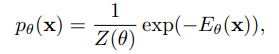Basically, EBM is trained to minimize this negative log-likelihood $-log(p_{\theta}(x))$. However, the distribution function $Z_{\theta}$ cannot be calculated analytically because it needs to be integrated over all $x$, and the density itself cannot be determined.

EBM training with neural networks requires gradients on the parameters of the negative log-likelihood, which are calculated as the difference between the expected values of the data distribution $p_{data}(x)$ and the model distribution $p_{\theta}$, respectively, as follows.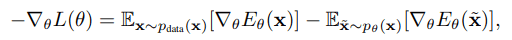In the above equation, sampling from the model distribution is difficult and MCMC is usually used. However, MCMC is known to have problems such as slow convergence when $x$ is high dimensional.

Using the Langevin Monte Carlo method, a variant of the Hamiltonian Monte Carlo method, sampling from the model distribution can be done as follows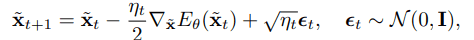EBM still faces various challenges such as stability of learning and diversity of generated data. In the next section, we introduce a method to solve these problems by taking advantage of the characteristics of the image data structure.

## Coarse-To-Fine EBM (CF-EBM)

In the image generation using EBM, the higher the image quality, the more difficult it is to sample from the model distribution due to the increased multimodality of the energy function. On the other hand, it is known that downsampled low-dimensional images change more smoothly than high-dimensional images in previous studies.

Based on these results, we expect that learning EBM from low-dimensional images is more stable and converges faster.

Coase-To-Fine EBM shifts the training from low quality to high quality by gradually adding layers to the neural network representing the energy function.

### Multi-step training using multiple resolution images

When moving the training from low to high resolution, we perform multi-step training using multi-resolution images generated from the training images. Let the number of training steps is $S$ and the image at each step $s$ be $x^{(s)}$. $x^{(s-1)}$ is generated by mean pooling on $x^{(s)}$, $x^{(0)}$ represents the lowest resolution image, and $x^{(S)}$ represents the training image.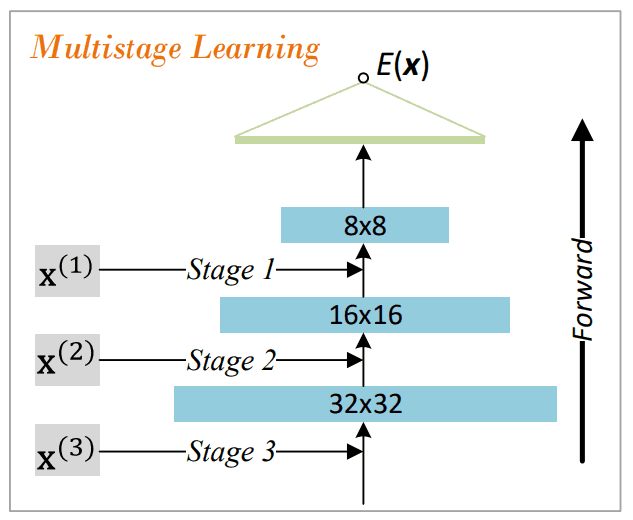The energy function $E^{(s)}$ learned in step s is transformed into a new energy function $E^{(s+1)}$ by adding a randomly initialized layer to the bottom layer when moving to the next step.

### Devices for stabilizing learning

To improve the stability of the above-mentioned multi-stage learning, two approaches are proposed in the paper concerning architecture and sampling.

### Smooth architecture changes

To avoid disturbing the energy function learned one step earlier when adding a randomly initialized layer to the bottom layer, we propose a smoothly changing architectural modification. Specifically, we define a layer (Expand block) that calculates the weighted sum of the outputs for two neural networks, Primal block and Fade block, and add it to the bottom layer.

$Expand(x)=\beta Primal(x) + (1 - \beta) Fade(x)$

As can be seen from the above equation, by gradually moving $\beta$ from 0 to 1, we can achieve learning that gradually focuses on detailed features.

### Smooth MCMC sampling

When the step moves from $s-1$ to $s$, $E^{(s-1)}$ and Fade blocks mainly contribute to the learning in the early stage. Then, as the learning progresses and the coefficient $\beta$ approaches 1, the Primal block is trained intensively. Therefore, the sampling by MCMC reflects the gradient of the Primal block as the training progresses, and a higher resolution image is sampled.

Furthermore, we initialize the data with noise added to the samples obtained in the energy function $E^{(s-1)}$ in the previous step to smooth the training transition.

## experimental results

Experiments of image generation, image restoration, anomaly detection, and domain transformation were conducted to evaluate CF-EBM. We will explain each experiment in detail.

### image generation

The performance evaluation of CF-EBM trained with CIFAR-10 is shown in the table below, where deep generative models such as VAE and GAN are used for the generation, and Frechet Inception Distance (FID) is set as the evaluation index. The smaller the FID, the better the index.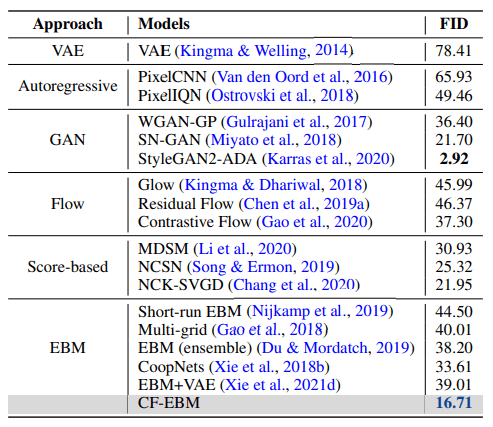As can be seen from the table, CF-EBM outperforms most of the other methods and records the best performance among the EBMs by a large margin.

The results when using CelebA as a dataset are shown in the table below, and as in the case of CIFAR-10, the evaluation was performed using FID.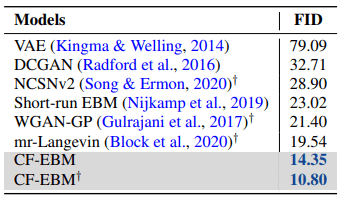Here too, CF-EBM outperforms the other methods. However, those marked with ♰ were tested using a resized image with the center hollowed out to a square.

### image restoration

Once the energy function has been learned, it can be used for image restoration tasks. In our paper, we performed two image restoration tasks: denoising and space completion.

For noise reduction, white noise was added to a randomly selected test image from CIFAR-10, and the image was restored by sampling with Langevin dynamics.

In addition, blank completion masked 25% of each image, and image restoration was performed on the masked areas by sampling with Langevin dynamics.

The two figures below show these results. The upper one is the result of denoising and the lower one is the result of space completion.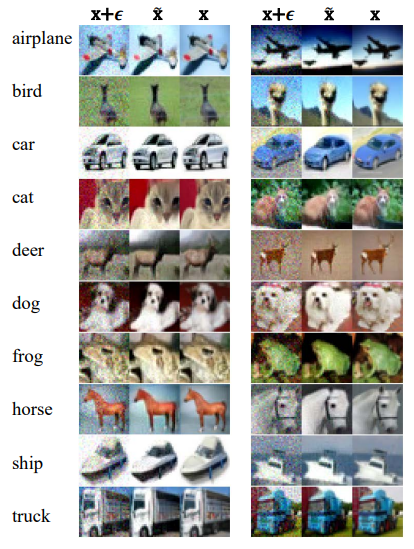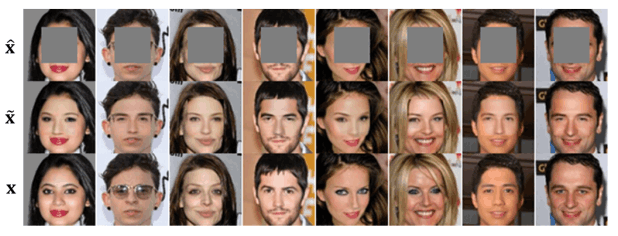In both experiments, the reconstructed images are similar to the original images in terms of general features but differ in terms of detailed features such as background color and the presence of glasses. This suggests that trained EBMs do not memorize training samples, but can also generate new samples.

### abnormal detection

Anomaly detection is a binary classification problem that solves whether a given data is normal or abnormal, and previous studies have shown that the likelihood of EBM is useful for anomaly detection.

The output of the energy function for normal data takes a large value, and the output of the energy function for abnormal data takes a small value.

Four deep generative models, including CF-EBM, were trained using CIFAR-10, with SVHN, uniform distribution, constant, linear interpolation between images, and CIFAR-100 samples as anomaly data. The results are shown in the table below. AUROC is used as the evaluation index.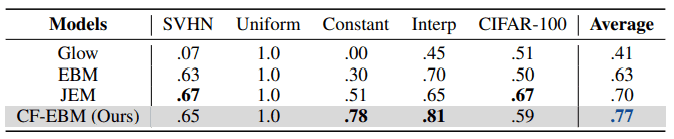As you can see in the table above, the average performance on the dataset for anomaly detection is the highest. It is also clear that CF-EBM performs well on anomalous data that can be easily mistaken for normal data, such as linear interpolation between images. Furthermore, considering that JEM uses image labels during training, while CF-EBM does not use labels, the performance of CF-EBM is not bad compared to CIFAR-100.

### domain translation

Domain transformation of an image is the task of transforming the style of colors and textures while preserving the content such as objects contained in the image.

The domain transformation using EBM is performed by initializing the sampling by the Langevin dynamics method with the source domain image and generating the target domain image. The target domain image is generated by initializing the sampling with the source domain image.

Conventional methods such as CycleGAN take into account the loss of constraint to return to the original image (Cycle consistency) when learning bi-directional domain transformations and transforming from source to target and target to source. However, the EBM-based domain transformation does not require this cycle consistency.

The figure below shows the results of the domain transformation on three datasets: orange-apple, cat-dog, and photo-Vangogh. It can be seen that we can generate more detailed images than other methods.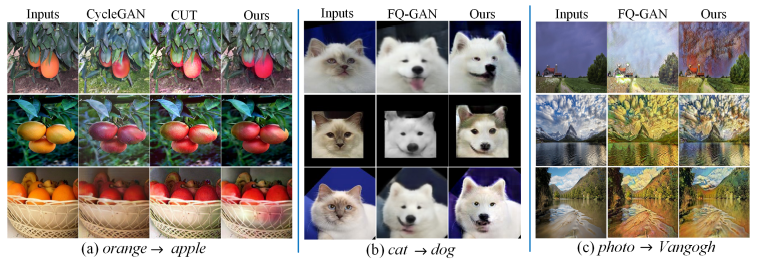## in the end

How did you like it? In this paper, we have improved the efficiency of sampling high-dimensional data in MCMC by "stepwise sampling from low resolution to high resolution ", which is a challenge in training EBM. It was also interesting to note that no cycle consistency is required when domain transformation of images is performed using EBM.

I hope that the scope of application of EBM will expand in the future. If you're interested, I urge you to read the original paper!

If you have any suggestions for improvement of the content of the article,